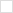This wiki is locked. Future workgroup activity and specification development must take place at our new wiki. For more information, see this blog post about the new governance model and this post about changes to the website.
TWiki>Main Web>PmHome>MetricsHome>MetricsWorkingDocuments>MetricsEMS10RestApi>MetricsEMS10RestApiDataModel>MetricsEmsMeasure (24 Sep 2010, ArthurRyman)

# ems:Measure

## Description

A measure is any quantity, such as cost, effort, duration, or size, that can be measured. Most measures are numeric quantities which may either take on discrete values or vary continuously. For example, defect count is a measure which takes on integer values, whereas duration can vary continuously. In practice, measurements are made at some level of accuracy, so even though a measure such as duration may vary continously, we can also measure it to some accuracy, e.g. days, hours, or even milliseconds. Furthermore, some discrete measures, such as code size measured in lines of code, take on vary large values, e.g. thousands or millions, so the fact that this measure is discrete is relevant. We therefore treat both the continuous and discrete cases as taking on continuous numeric values, which we represent used the datatype xsd:double.

The quantity being measured is referred to as a metric. A given metric may be measured using one or more units of measure. For example, effort is a metric and it may be measured in person-hours, person-months, or person-years. We use URIs to identify metrics and units of measure.

The ems:Measure resource represents a measure.

### Measuring Time

In the course of a project it is useful to measure when certain events occur. For example, project start and finish dates are key metrics. However, we do not usually think of a point in time as a numeric value since we typically identity points in time using a special date-time format such as xsd:dateTime. When use examine date-time formats more closely when see in fact that they do measure the quantity of time that has passed since some reference point.

For example, an xsd:dateTime such as 2010-04-08 identifies the point in time that occurred 2010 years, 4 months, and 8 days after a historical reference point defined by the Gregorian calendar. We can convert this date-time into a numeric quantity that measures the elapsed time from the Gregorian reference point measured in milliseconds, seconds, minutes, hours, days, weeks, years, decades, centuries, etc. In doing doing we must define the conversion factors between these units of measure.

An alternate system for measuring time is UNIX time, also known as POSIX time, which uses 1970-01-01, the so-called Epoch, as the reference point, and uses seconds as the unit of measure. For example, UNIX time 1234567890 is Gregorian time 2009-02-13T23:31:30. Computer systems provide functions for converting between UNIX time and Gregorian time.

In principle, we could be flexible and allow time measurements to be identified in either xsd:dateTime or xsd:double. However, measure values occur in many resource representations, e.g. probabililty distriutions and measure cells. Allowing both xsd:dateTime and xsd:double in all these resources would add a lot of complexity. Therefore, for simplicity, we uniformly use only xsd:demical whenever we deal with measures. This means that time measures MUST use some numeric unit of measure, such as UNIX time, that can be represented as xsd:double.

## Properties

Property Range Type Occurrence Edits Description
dcterms:title datatype rdf:XMLLiteral exactly-one read-write A brief title for the measure. Valid XHTML <span> element content.
ems:metric resource rdf:Resource exactly-one read-write A URI that identifies the metric.
ems:unitOfMeasure resource rdf:Resource exactly-one read-write A URI that identifies the unit of measure.
ems:numericValue datatype xsd:double exactly-one read-write The numeric value of the measure.

### dcterms:title

This required property gives a short, human-readable title for the measure. Its datatype is XML literal and it MUST contain valid XHTML <span> element content.

### ems:metric

This requried property gives a URI that identifies the metric. See Software Metrics Definitions for standard URIs.

### ems:unitOfMeasure

This required property gives a URI that identifies the unit of measure. See Software Metrics Definitions for standard URIs.

### ems:numericValue

This required property gives the numeric value of the measure.

## Examples

### Measure 1

The following listing contains an ems:Measure resource that describes an observation of total project duration with an actual value of 6 months.

###### Listing of Measure 1
```<?xml version="1.0"?>
<ems:Measure xmlns:ems="http://open-services.net/ns/ems#"
xmlns:rdf="http://www.w3.org/1999/02/22-rdf-syntax-ns#" xmlns:dcterms="http://purl.org/dc/terms/">
<dcterms:title rdf:parseType="Literal">Total Duration in Months</dcterms:title>
<ems:metric rdf:resource="http://open-services.net/ns/ems/metric#Duration" />
<ems:unitOfMeasure
rdf:resource="http://open-services.net/ns/ems/unit#Month" />
<ems:numericValue rdf:datatype="http://www.w3.org/2001/XMLSchema#double">6</ems:numericValue>
</ems:Measure>

```Български Cesky Dansk Deutsch English Español Français Italiano 日本語 Nederlands Polski Português Русский Svenska 简体中文 簡體中文